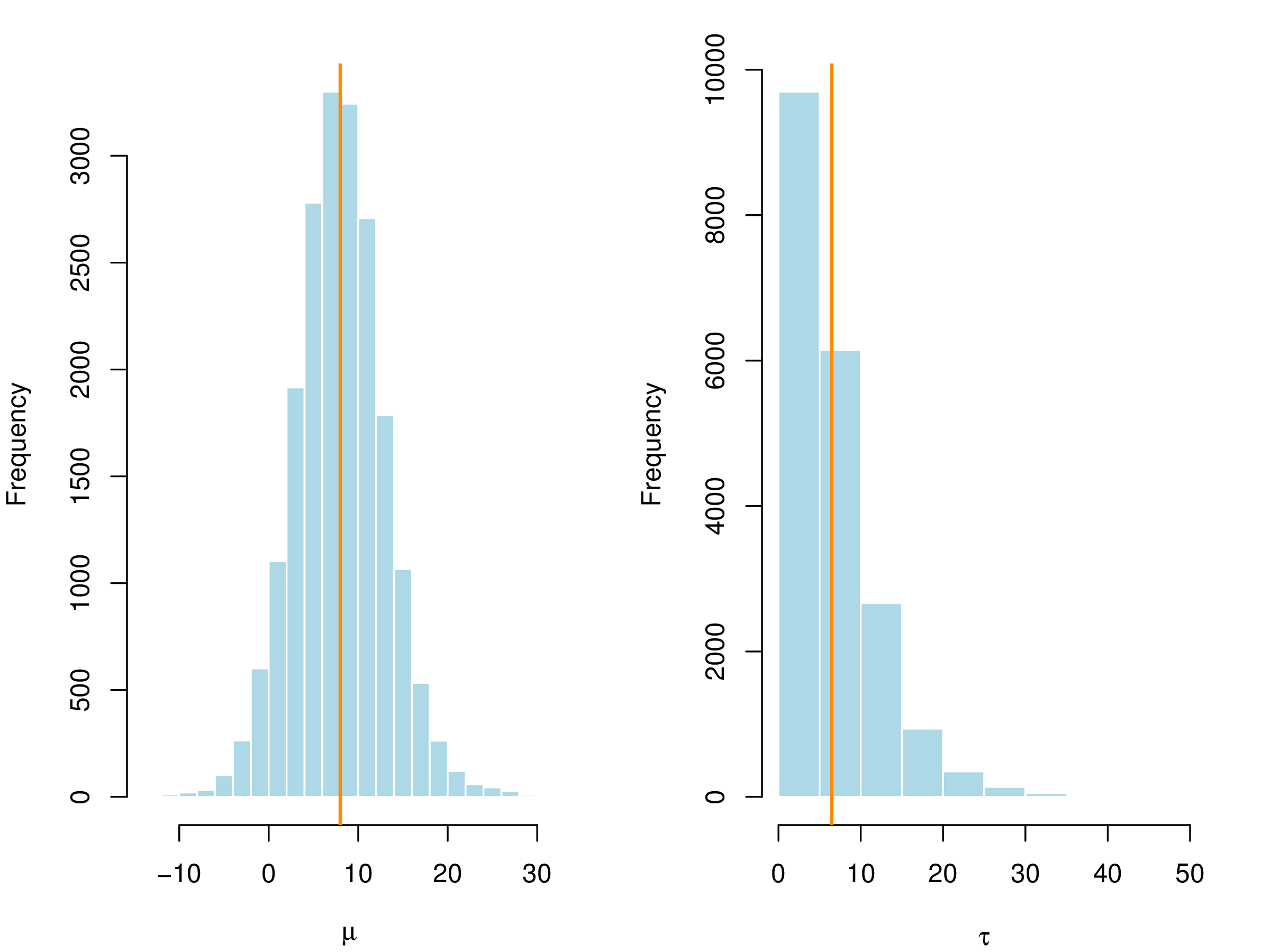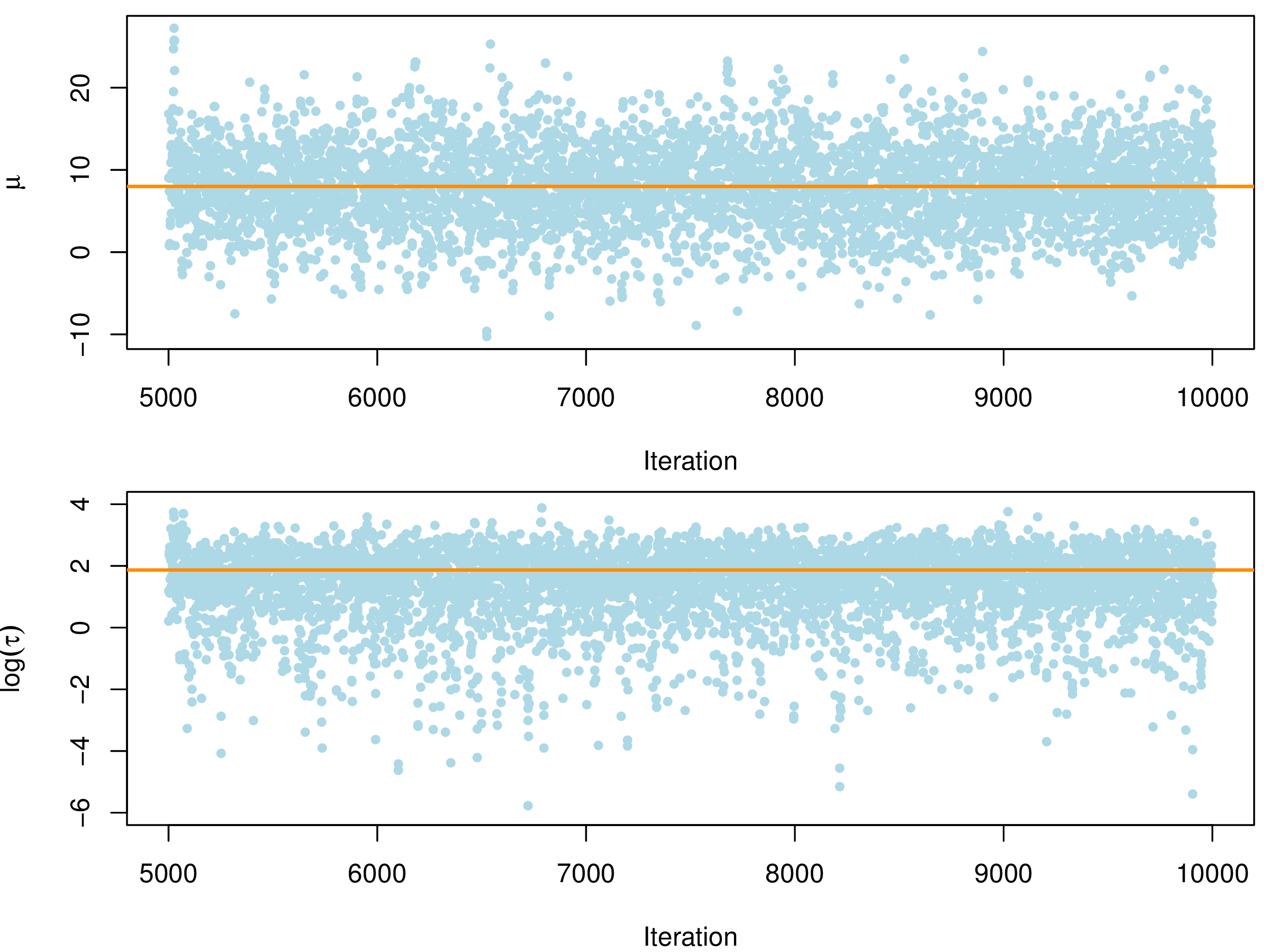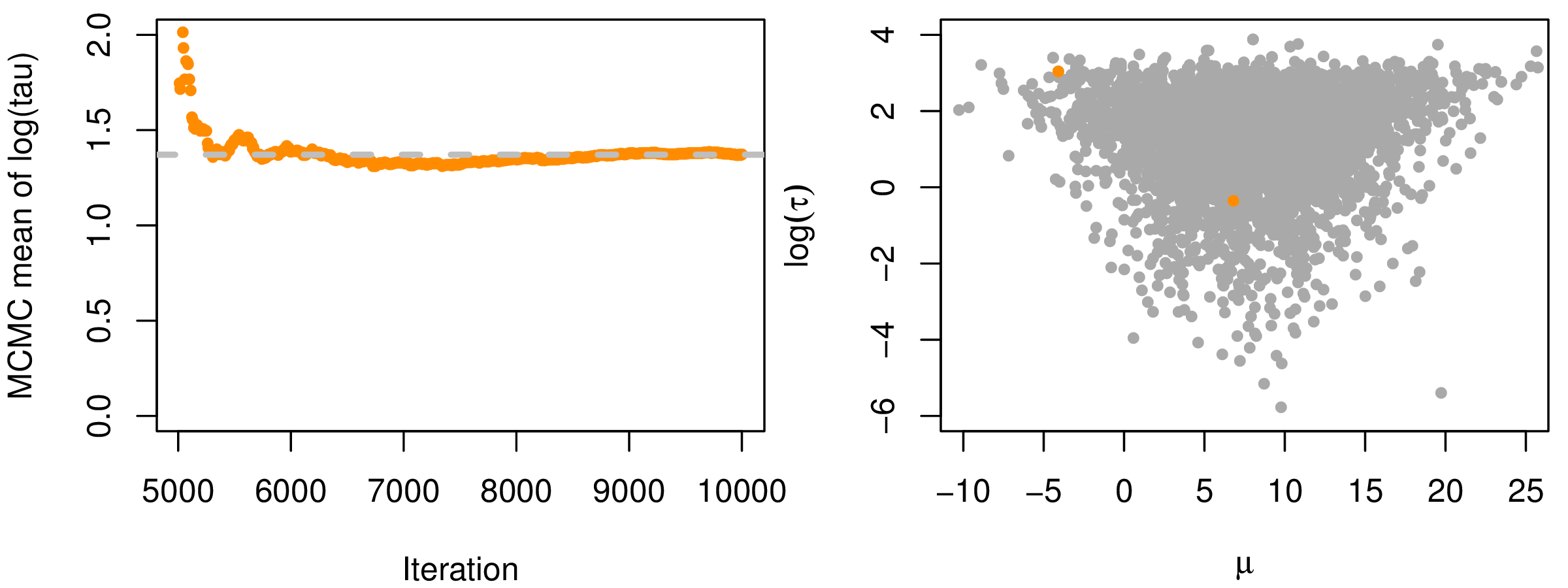# 第 4 章 参数估计

4.5 节详细介绍在贝叶斯 Langevin-Hastings 算法的基础上提出基于 Stan 实现的汉密尔顿蒙特卡罗算法（简称 Stan-HMC 算法），并分四个小节进行，第4.5.2小节介绍算法提出的背景和意义，第 4.5.3 小节从 Stan 的发展、内置算法设置以及与同类软件的比较等三方面介绍，然后以数据集 Eight Schools 为例子介绍 Stan 的使用，为空间广义线性混合效应模型的 Stan 实现作铺垫，第4.5.4小节介绍 Stan-HMC 算法实现过程。

## 4.1 极大似然估计

$\begin{equation} L(\boldsymbol{\psi};\mathbf{y}) = \int \prod_{i=1}^{n} f(y_i|u_i;\boldsymbol{\beta})\phi_{n}(\mathbf{u};0,\Sigma_{\boldsymbol{\theta}})\mathrm{d}\mathbf{u} \tag{4.1} \end{equation}$

\begin{equation} \begin{aligned} \pi(\boldsymbol{\psi},\mathbf{u}|\mathbf{y}) &= \frac{f(\mathbf{y|\mathbf{u}, \boldsymbol{\psi}})\phi_{n}(\mathbf{u};0,\Sigma_{\boldsymbol{\theta}})\pi(\boldsymbol{\psi})}{m(\mathbf{y})} \\ m(\mathbf{y}) &= \int f(\mathbf{y|\mathbf{u}, \boldsymbol{\psi}})\phi_{n}(\mathbf{u};0,\Sigma_{\boldsymbol{\theta}})\pi(\boldsymbol{\psi})\mathrm{d} \mathbf{u} \mathrm{d} \boldsymbol{\psi} \end{aligned} \end{equation}

## 4.2 剖面似然估计

1. 重参数化 $$\nu^2 = \tau^2/\sigma^2$$，令 $$V = \mathbf{R}(\phi) + \nu^2 \mathbf{I}$$
2. 给定 $$V$$，对数似然函数 (4.3)\begin{equation} \begin{aligned} \hat{\boldsymbol{\beta}}(V) & = (D^{\top} V^{-1} D)^{-1} D^{\top} V^{-1}\mathbf{Y} \\ \hat{\sigma}^2(V) & = n^{-1} \{\mathbf{Y} - D\hat{\boldsymbol{\beta}}(V)\}^{\top} V^{-1} \{\mathbf{Y} - D\hat{\boldsymbol{\beta}}(V)\} \end{aligned} \tag{4.4} \end{equation} 取得极大值；
3. (4.4) 式代入对数似然函数 (4.3) 式，可获得一个简化的对数似然 $\begin{equation} L_{0}(\nu^2,\phi) = - 0.5\{ n\log(2\pi) + n\log \hat{\sigma}^2(V) + \log |V| + n \} \tag{4.5} \end{equation}$
4. 关于参数 $$\nu^2, \phi$$ 极大化 (4.5) 式，获得参数 $$\nu^2, \phi$$ 的估计值，再将其回代 (4.4) 式，获得估计值 $$\hat{\boldsymbol{\beta}}$$$$\hat{\sigma}^2$$

## 4.4 参数估计的算法

### 4.4.1 拉普拉斯近似极大似然算法

\begin{equation} \begin{aligned} \mathbf{Y(x)} | S(\mathbf{x}) & \sim f(\cdot;\boldsymbol{\mu(x)},\psi) \\ g(\boldsymbol{\mu}(\mathbf{x})) & = D\boldsymbol{\beta} + S(\mathbf{x}) = D\boldsymbol{\beta} + \sigma R(\mathbf{x};\phi) + \tau z \\ S(\mathbf{x}) & \sim \mathcal{N}(\mathbf{0},\Sigma) \end{aligned} \tag{4.7} \end{equation}

SGLMM 模型假定在给定高斯空间过程 $$S(\mathbf{x})$$ 的条件下， $$Y_1,Y_2,\ldots,Y_n$$ 是独立的，并且服从分布 $$f(\cdot;\boldsymbol{\mu}(\mathbf{x}),\psi)$$。此分布的参数有两个来源，其一是与联系函数 $$g$$ 关联的线性预测 $$\boldsymbol{\mu}(\mathbf{x})$$，其二是密度分布函数 $$f$$ 的发散参数 $$\psi$$，可以看作是似然函数中的附加参数。空间过程 $$S(\mathbf{x})$$ 分解为空间相关 $$R(\mathbf{x};\phi)$$ 和独立过程 $$Z$$，二者分别被参数 $$\sigma$$$$\tau$$ 归一化而具有单位方差。线性预测包含一组固定效应 $$D\boldsymbol{\beta}$$，空间相关的随机效应 $$R(\mathbf{x};\phi)$$，与空间不相关的随机效应 $$\tau z \sim \mathcal{N}(\mathbf{0},\tau^2\mathbf{I})$$$$D$$ 是根据协变量观测值得到的数据矩阵，$$\boldsymbol{\beta}$$$$p \times 1$$ 维的回归参数向量。

$$R(\mathbf{x};\phi)$$ 是具有单位方差的平稳空间高斯过程，其自相关函数为 $$\rho(u,\phi)$$，这里 $$u$$ 表示一对空间位置之间的距离，$$\phi$$ 是刻画空间相关性的参数。自相关函数 $$\rho(u,\phi) (\in \mathbb{R}^d)$$$$d$$ 维空间到一维空间的映射函数，特别地，假定空间过程 $$S(\mathbf{x})$$的自相关函数仅仅依赖成对点之间的欧氏距离，即 $$u =\|x_i - x_j\|$$。常见的自相关函数有指数型、梅隆型和球型。线性预测的随机效应部分协方差矩阵 $$\Sigma = \sigma^2 R(\mathbf{x};\phi) + \tau^2\mathbf{I}$$

$\begin{equation} L(\boldsymbol{\theta};\mathbf{y}) = \int_{\mathbb{R}^n} [\mathbf{Y(x)}|S(\mathbf{x})][S(\mathbf{x})]\mathrm{d}S(\mathbf{x}) \tag{4.8} \end{equation}$

$\begin{equation} I = \int_{\mathbb{R}^n} \exp\{Q(\mathbf{s})\}\mathrm{d}\mathbf{s} \approx (2\pi)^{n/2} |-Q''(\hat{\mathbf{s}})|^{-1/2}\exp\{Q(\hat{\mathbf{s}})\} \tag{4.9} \end{equation}$

$\begin{equation} f(\mathbf{y};\boldsymbol{\beta}) = \exp\{\mathbf{y}^{\top} (D\boldsymbol{\beta} + S(\mathbf{x})) - \mathbf{1}^{\top} b( D\boldsymbol{\beta} + S(\mathbf{x})) + \mathbf{1}^{\top} c(\mathbf{y}) \} \tag{4.10} \end{equation}$

\begin{align} f(S(\mathbf{x});\Sigma) & = (2\pi)^{-n/2}|\Sigma|^{-1/2} \exp\{ -\frac{1}{2}S(\mathbf{x})^{\top} \Sigma^{-1} S(\mathbf{x}) \} \\ & = \exp\{ - \frac{n}{2}\log (2\pi) -\frac{1}{2}\log |\Sigma| -\frac{1}{2}S(\mathbf{x})^{\top} \Sigma^{-1} S(\mathbf{x}) \} \tag{4.11} \end{align}

$\begin{equation} L(\boldsymbol{\theta};\mathbf{y}) = \int_{\mathbb{R}^n} \exp\{Q(S(\mathbf{x}))\} \mathrm{d}S(\mathbf{x}) \end{equation}$

\begin{equation} \begin{aligned} Q(S(\mathbf{x})) ={} & \mathbf{y}^{\top} (D \boldsymbol{\beta} + S(\mathbf{x})) - \mathbf{1}^{\top} b(D \boldsymbol{\beta} + S(\mathbf{x})) + \mathbf{1}^{\top}c(\mathbf{y}) \\ & - \frac{n}{2}\log (2\pi) -\frac{1}{2}\log |\Sigma| -\frac{1}{2}S(\mathbf{x})^{\top} \Sigma^{-1} S(\mathbf{x}) \end{aligned} \tag{4.12} \end{equation}

$\begin{equation} \mathbf{s}_{i+1} = \mathbf{s}_{i} - Q''(\mathbf{s}_{i})^{-1}Q'(\mathbf{s}_{i}) \end{equation}$

\begin{align} Q'(\mathbf{s})& = \{\mathbf{y} - b'(D\boldsymbol{\beta} + \mathbf{s}) \}^{\top} - \mathbf{s}^{\top}\Sigma^{-1} \tag{4.13} \\ Q''(\mathbf{s})& = -\mathrm{diag} \{b''(D\boldsymbol{\beta} + \mathbf{s}) \} - \Sigma^{-1} \tag{4.14} \end{align}

\begin{equation} \begin{aligned} \ell(\boldsymbol{\theta};\mathbf{y}) = {} & \frac{n}{2}\log (2\pi) -\frac{1}{2}\log | -\mathrm{diag} \{b''(D\boldsymbol{\beta} + \mathbf{s}) \} - \Sigma^{-1} | \\ & + \mathbf{y}^{\top} (D\boldsymbol{\beta} + \hat{\mathbf{s}}) - \mathbf{1}^{\top} b( D\boldsymbol{\beta} + \hat{\mathbf{s}}) + \mathbf{1}^{\top} c(\mathbf{y}) \\ & - \frac{n}{2}\log (2\pi) -\frac{1}{2}\log |\Sigma| -\frac{1}{2}\hat{\mathbf{s}}^{\top} \Sigma^{-1} \hat{\mathbf{s}} \end{aligned} \tag{4.15} \end{equation}

$\hat{\boldsymbol{\theta}} \sim \mathcal{N}(\boldsymbol{\theta}, \mathbf{I}_{o}^{-1}(\hat{\boldsymbol{\theta}}))$

1. 选择模型参数 $$\boldsymbol{\theta}$$ 的初始值 $$\boldsymbol{\theta}_{i}$$
2. 计算协方差矩阵 $$\Sigma$$ 及其逆 $$\Sigma^{-1}$$
3. 通过如下步骤极大$$Q$$函数，获得估计值 $$\hat{\mathbf{s}}$$
1. $$\mathbf{s}$$ 选择初始值；
2. (4.13) 式计算 $$Q'(\mathbf{s})$$，按 (4.14) 式计算 $$Q''(\mathbf{s})$$，其中导数计算的代码实现可参考黄湘云（2016年） (黄湘云 2016)
3. 解线性方程组 $$Q''(\mathbf{s})\mathbf{s}^{\star} = Q'(\mathbf{s})$$
4. 更新 $$\mathbf{s = s + s^{\star}}$$
5. 迭代直到收敛以获得 $$\hat{\mathbf{s}}$$
4. $$\hat{\mathbf{s}}$$ 替换 $$S(\mathbf{x})$$，在 (4.12) 式中计算 $$Q(\hat{\mathbf{s}})$$
5. (4.9) 式计算积分的近似值，以获得边际似然 (4.15) 式的值；
6. 用 BFGS 算法获得下一个值 $$\boldsymbol{\theta}_{i+1}$$
7. 重复上述过程直到收敛，获得参数的估计值 $$\hat{\boldsymbol{\theta}}$$

NR算法收敛速度是很快的，但是必须提供一个很好的初值，好的初值对于快速收敛到似然函数 $$\ell(\boldsymbol{\theta};\mathbf{y})$$ 的极大值点很重要。指定外迭代中的初值 $$\boldsymbol{\theta}_{0}$$的一个策略是首先拟合一个简单的广义线性模型，获得回归系数 $$\boldsymbol{\beta}$$ 的初值，基于这些值计算线性预测值 $$\hat{\boldsymbol{\mu}}$$；然后计算残差 $$\hat{\boldsymbol{r}} = (\hat{\boldsymbol{\mu}} - \mathbf{y})$$$$\hat{\boldsymbol{r}}$$ 的方差作为 $$\sigma^2$$ 的初值，如果 SGLMM 带有块金效应，就用 $$\sigma^2$$ 的初值的一定比例，如 10% 作为 $$\tau^2$$ 的初值；最后，$$\phi$$ 的初值选择两个距离最大的观测点之间的距离的 10%，比较保险的办法是选择不同的 $$\phi$$ 作为初值，这个过程需要不断的试错以期获得算法的收敛(Bonat and Ribeiro Jr. 2016)

### 4.4.2 蒙特卡罗极大似然算法

$\begin{equation} g(\mu_i) = T_{i} = d(x_i)^{\top}\boldsymbol{\beta} + S(x_i) + Z_i \tag{4.16} \end{equation}$

$\begin{equation} L(\boldsymbol{\beta},\boldsymbol{\theta}) = f(y;\boldsymbol{\beta},\boldsymbol{\theta}) = \int_{\mathbb{R}^{n}}\mathcal{N}(t;D\boldsymbol{\beta},\Sigma(\boldsymbol{\theta}))f(y|t)dt \tag{4.19} \end{equation}$

\begin{equation} \begin{aligned} L(\boldsymbol{\beta},\boldsymbol{\theta}) & = \int_{\mathbb{R}^{n}} \frac{\mathcal{N}(t;D\boldsymbol{\beta},\Sigma(\boldsymbol{\theta}))f(y|t)}{\mathcal{N}(t;D\boldsymbol{\beta}_{0},\Sigma(\boldsymbol{\theta}_{0}))f(y|t)}f(y,t)dt \\ & \varpropto \int_{\mathbb{R}^{n}} \frac{\mathcal{N}(t;D\boldsymbol{\beta}, \Sigma(\boldsymbol{\theta}))}{\mathcal{N}(t;D\boldsymbol{\beta}_{0}, \Sigma(\boldsymbol{\theta}_{0}))}f(t|y)dt \\ &= E_{T|y}\left[\frac{\mathcal{N}(t; D\boldsymbol{\beta}, \Sigma(\boldsymbol{\theta}))}{\mathcal{N}(t; D\boldsymbol{\beta}_{0}, \Sigma(\boldsymbol{\theta}_{0}))}\right] \end{aligned} \tag{4.20} \end{equation}

$\begin{equation} L_{m}(\boldsymbol{\beta},\boldsymbol{\theta})=\frac{1}{m}\sum_{i=1}^{n}\frac{\mathcal{N}(t_{i};D\boldsymbol{\beta},\Sigma(\boldsymbol{\theta}))}{\mathcal{N}(t_{i};D\boldsymbol{\beta}_{0},\Sigma(\boldsymbol{\theta}_{0}))} \tag{4.21} \end{equation}$

$\max_{\boldsymbol{\beta},\boldsymbol{\theta}}L_{m}(\boldsymbol{\beta},\boldsymbol{\theta}) \rightarrow 1, m \rightarrow\infty$

### 4.4.3 贝叶斯 Langevin-Hastings 算法

$\begin{equation} \pi(\boldsymbol{\beta}, \boldsymbol{\theta}, t | y) \propto g(\boldsymbol{\theta})\mathcal{N}(\boldsymbol{\beta}; \xi, \sigma^2 \Omega)\mathcal{N}(t; D\boldsymbol{\beta}, \Sigma(\boldsymbol{\theta}))f(y|t) \tag{4.22} \end{equation}$

R 包 PrevMap 内的函数 binomial.logistic.Bayes 可以从上述后验分布中抽得样本，这个抽样的过程使用了 MCMC 算法， $$\boldsymbol{\theta}, \boldsymbol{\beta}$$$$\mathbf{T}$$ 轮流迭代的过程如下：

1. 初始化$$\boldsymbol{\beta}, \boldsymbol{\theta}$$$$\mathbf{T}$$
2. 对协方差$$\Sigma(\boldsymbol{\theta})$$中的参数做如下变换 (Christensen, Roberts, and Sköld 2006) $(\tilde{\theta}_{1}, \tilde{\theta}_{2}, \tilde{\theta}_{3}) = (\log \sigma, \log (\sigma^2/\phi^{2\kappa}), \log \tau^2)$ 使用随机游走 Metropolis-Hastings 算法轮流更新上述三个参数，在第 $$i$$ 次迭代时，候选高斯分布的标准差 $$h$$$$h_{i} = h_{i-1} + c_{1}i^{-c_{2}}(\alpha_{i}-0.45)$$，其中，$$c_{1} > 0$$$$c_{2} \in (0,1]$$ 是预先给定的常数，$$\alpha_i$$ 是第 $$i$$ 次迭代时的接受概率，其中 0.45 是一元高斯分布的最优接受概率；
3. 使用Gibbs步骤更新 $$\boldsymbol{\beta}$$， 所需条件分布 $$\boldsymbol{\beta}|\boldsymbol{\theta},\mathbf{T}$$ 是高斯分布，均值 $$\tilde{\xi}$$，协方差矩阵 $$\sigma^2\tilde{\Omega}$$，且与 $$y$$ 不相关，记$$\Sigma(\boldsymbol{\theta}) = \sigma^2 R(\boldsymbol{\theta})$$ $\tilde{\xi} = \tilde{\Omega}(\Omega^{-1}\xi+D^{\top} R(\boldsymbol{\theta})^{-1} \mathbf{T}), \quad \sigma^2 \tilde{\Omega} = \sigma^2(\Omega^{-1} + D^{\top} R(\boldsymbol{\theta})^{-1} D)^{-1}$
4. 使用汉密尔顿蒙特卡罗算法更新条件分布 $$\mathbf{T}|\boldsymbol{\beta},\boldsymbol{\theta},y$$，用 $$H(t,u)$$ 表示汉密尔顿函数 $H(t, u) = u^{\top} u/2 - \log f(t | y, \boldsymbol{\beta}, \boldsymbol{\theta})$ 其中，$$u\in\mathbb{R}^2$$$$f(t | y, \boldsymbol{\beta}, \boldsymbol{\theta})$$ 表示给定 $$\boldsymbol{\beta}$$$$\boldsymbol{\theta}$$$$y$$下，$$\mathbf{T}$$ 的条件分布。根据汉密尔顿方程，函数 $$H(u, t)$$ 的偏导决定 $$u,t$$ 随时间 $$v$$ 的变化过程， $\begin{eqnarray*} \frac{d t_{i}}{d v} & = & \frac{\partial H}{\partial u_{i}} \\ \frac{d u_{i}}{d v} & = & -\frac{\partial H}{\partial t_{i}} \end{eqnarray*}$ 其中，$$i = 1,\ldots, n$$， 上述动态汉密尔顿微分方程根据 leapfrog 方法(Brooks et al. 2011)离散， 然后求解离散后的方程组获得近似解。

### 4.4.4 低秩近似算法

$\begin{equation} \log\{\frac{p_i}{1-p_i}\} = T_{i} = d(x_i)^{\top}\boldsymbol{\beta} + S(x_i) + Z_{i} \tag{4.27} \end{equation}$

$\begin{equation} S(x) = \int_{\mathbb{R}^2} K(\|x-t\|; \phi, \kappa) \: d B(t) \tag{4.28} \end{equation}$

$\begin{equation} K(u; \phi, \kappa) = \frac{\Gamma(\kappa + 1)^{1/2}\kappa^{(\kappa+1)/4}u^{(\kappa-1)/2}}{\pi^{1/2}\Gamma((\kappa+1)/2)\Gamma(\kappa)^{1/2}(2\kappa^{1/2}\phi)^{(\kappa+1)/2}}\mathcal{K}_{\kappa}(u/\phi), u > 0 \tag{4.29} \end{equation}$

$\begin{equation} S(x) \approx \sum_{i = 1}^r K(\|x-\tilde{x}_{i}\|; \phi, \kappa) U_{i} \tag{4.30} \end{equation}$

(4.30)中， $$(\tilde{x}_{1},\ldots,\tilde{x}_{r})$$ 表示空间网格的格点，$$U_{i}$$ 是独立同分布的高斯变量，均值为$$0$$， 方差为$$\sigma^2$$。 特别地， 尺度参数$$\phi$$越大时，空间曲面越平缓，如图 3.3所示，在格点数 $$r$$ 比较少时也能得到不错的近似效果。 此外， 空间格点数 $$r$$ 与样本量 $$n$$ 是独立的， 因此， 低秩近似算法在样本量比较大时， 计算效率还比较高。

## 4.5 贝叶斯 Stan-HMC 算法

### 4.5.1 蒙特卡罗积分

4.1 列出了前 10 维超球的体积，从上述计算过程中，我们发现随着维数增加，超球的体积迅速变小。这里有一个反直观的现象，内切球的体积竟然随着维数的增加变小，并且在 10 维的情形下，内切球的体积已不到超立方体的 0.3%，可以预见如果这个积分是 100 维甚至更多，那么内切球相比于正方体仅仅是一个极小的角落，随机点会越来越难以落在内切球内。甚至会因为所需要的随机数太多或者计算机资源的限制，而不可计算，开发更加高效的随机模拟算法也就势在必行。

### 4.5.3 Stan 简介

Stan 内置的采样器 No-U-Turn （简称 NUTS） 源于汉密尔顿蒙特卡罗算法 （Hamiltonian Monte Carlo，简称 HMC），最早由 Hoffman 和 Gelman （2014年） (Hoffman and Gelman 2014) 提出。与 Stan 有相似功能的软件 BUGS 和 JAGS 主要采用的是 Gibbs 采样器，前者基于 Pascal 语言开发于 1989 年至 2004 年，后者基于 C++ 活跃开发于 2007 年至 2013 年。在时间上， Stan 具有后发优势，特别在灵活性和扩展性方面，它支持任意的目标函数，模型语言也更加简单易于推广学习，其每一行都是命令式的语句，而 BUGS 和 JAGS 采用声明式；在大量数据的建模分析中， Stan 可以更快地处理复杂模型，这一部分归功于它高效的算法实现和内存管理，另一部分在于高级的 MCMC 算法 — 带 NUTS 采样器的 HMC 算法。

Rubin （1981年） (Rubin 1981) 分析了 Alderman 和 Powers (Alderman and Powers 1980) 收集的原始数据，得出表 4.2， Gelman 和 Carlin 等 （2003年） (Gelman et al. 2003) 建立分层正态模型 (4.31) 分析 Eight Schools 数据集，由美国教育考试服务调查搜集，用以分析不同的培训项目对学生考试分数的影响，其随机调查了 8 所高中，学生的成绩作为培训效应的估计 $$y_j$$，其样本方差 $$\sigma^2_j$$，数据集见表 4.2。这里再次以该数据集和模型为例介绍 Stan 的使用。

\begin{equation} \begin{aligned} &{} \mu \sim \mathcal{N}(0,5), \quad \tau \sim \text{Half-Cauchy}(0,5), \quad p(\mu,\tau) \propto 1 \\ &{} \eta_i \sim \mathcal{N}(0,1), \quad \theta_i = \mu + \tau \cdot \eta_i, \quad y_i \sim \mathcal{N}(\theta_i,\sigma^2_{i}), i = 1,\ldots,8 \end{aligned} \tag{4.31} \end{equation}

School A B C D E F G H
$$y_i$$ 28 8 -3 7 -1 1 18 12
$$\sigma_i$$ 15 10 16 11 9 11 10 18

mean se_mean sd 2.5% 25% 50% 75% 97.5% n_eff Rhat
$$\mu$$ 7.99 0.05 5.02 -1.65 4.75 7.92 11.15 18.10 8455 1
$$\tau$$ 6.47 0.06 5.44 0.22 2.45 5.18 9.07 20.50 7375 1
$$\eta_1$$ 0.40 0.01 0.93 -1.49 -0.21 0.42 1.02 2.19 16637 1
$$\eta_2$$ 0.00 0.01 0.87 -1.73 -0.58 0.00 0.57 1.70 16486 1
$$\eta_3$$ -0.20 0.01 0.93 -1.99 -0.82 -0.20 0.41 1.66 20000 1
$$\eta_4$$ -0.04 0.01 0.88 -1.80 -0.60 -0.04 0.53 1.74 20000 1
$$\eta_5$$ -0.36 0.01 0.88 -2.06 -0.94 -0.38 0.20 1.42 15489 1
$$\eta_6$$ -0.22 0.01 0.90 -1.96 -0.82 -0.23 0.37 1.57 20000 1
$$\eta_7$$ 0.34 0.01 0.89 -1.49 -0.24 0.36 0.93 2.04 16262 1
$$\eta_8$$ 0.05 0.01 0.94 -1.81 -0.57 0.06 0.69 1.91 20000 1
$$\theta_1$$ 11.45 0.08 8.27 -1.86 6.07 10.27 15.50 31.68 11788 1
$$\theta_2$$ 7.93 0.04 6.15 -4.45 3.99 7.90 11.74 20.44 20000 1
$$\theta_3$$ 6.17 0.06 7.67 -11.17 2.07 6.74 10.89 19.94 16041 1
$$\theta_4$$ 7.66 0.05 6.51 -5.63 3.75 7.72 11.62 20.78 20000 1
$$\theta_5$$ 5.13 0.05 6.41 -9.51 1.37 5.66 9.43 16.41 20000 1
$$\theta_6$$ 6.14 0.05 6.66 -8.63 2.35 6.58 10.40 18.47 20000 1
$$\theta_7$$ 10.64 0.05 6.76 -1.14 6.11 10.11 14.52 25.88 20000 1
$$\theta_8$$ 8.42 0.06 7.86 -7.24 3.91 8.26 12.60 25.24 16598 1
lp__ -39.55 0.03 2.64 -45.41 -41.15 -39.31 -37.67 -35.12 6325 1

4.3 的列为后验量的估计值：依次是后验均值 $$\mathsf{E}(\mu|Y)$$、 蒙特卡罗标准误（Monte Carlo standard error）、后验标准差 （standard deviation） $$\mathsf{E}(\sigma|Y)$$ 、后验分布的 5 个分位点、有效样本数 $$n_{eff}$$ 和潜在尺度缩减因子 （potential scale reduction factor），最后两个量 用来分析采样效率和评估迭代序列的平稳性；最后一行表示每次迭代的未正则的对数后验密度 （unnormalized log-posterior density） $$\hat{R}$$，当链条都收敛到同一平稳分布的时候，$$\hat{R}$$ 接近 1。\begin{equation} \begin{aligned} & B = \frac{n}{m-1}\sum_{j=1}^{m}(\bar{\omega}_{.j} - \bar{\omega}_{..} ), \quad \bar{\omega}_{.j} = \frac{1}{n}\sum_{i=1}^{n}\omega_{ij}, \quad \bar{\omega}_{..} = \frac{1}{m}\sum_{j=1}^{m} \bar{\omega}_{.j}\\ & W = \frac{1}{m}\sum_{j=1}^{m}s^{2}_{j}, \quad s^{2}_{j} = \frac{1}{n-1}\sum_{i=1}^{n}(\omega_{ij} - \bar{\omega}_{.j})^2 \end{aligned} \tag{4.32} \end{equation}

$$\omega$$ 的后验方差 $$\widehat{\mathsf{Var}}^{+}(\omega|Y)$$$$W$$$$B$$ 的加权平均

$\begin{equation} \widehat{\mathsf{Var}}^{+}(\omega|Y) = \frac{n-1}{n} W + \frac{1}{n} B \end{equation}$

$\begin{equation} \hat{R} = \sqrt{\frac{\widehat{\mathsf{Var}}^{+}(\omega|Y)}{W}} \end{equation}$

### 4.5.4 实现过程

1. 选择初始值 $$\boldsymbol{\theta},\boldsymbol{\beta},S$$，如 $$\boldsymbol{\beta}$$ 的初始值来自正态分布，$$\boldsymbol{\theta}$$ 的初始值来自对数正态分布；
2. 更新参数向量 $$\boldsymbol{\theta}$$
1. 从指定的先验分布中均匀抽取新的 $$\boldsymbol{\theta}'$$
2. 以概率 $$\Delta(\boldsymbol{\theta},\boldsymbol{\theta}') = \min \big\{\frac{p(S|\boldsymbol{\theta}')}{p(S|\boldsymbol{\theta})},1\big\}$$接受$$\boldsymbol{\theta}'$$，否则不改变 $$\boldsymbol{\theta}$$
3. 更新高斯过程 $$S$$ 的取值：
1. 抽取新的值 $$S_{i}'$$， 向量 $$S$$ 的第 $$i$$ 值来自一元条件高斯密度 $$p(S_{i}'|S_{-i},\boldsymbol{\theta})$$$$S_{-i}'$$ 表示移除 $$S$$ 中的第 $$i$$ 个值；
2. 以概率 $$\Delta(S_{i},S_{i}') = \min\big\{ \frac{p(y_{i}|s_{i}',\boldsymbol{\beta})}{p(y_{i}s_{i},\boldsymbol{\beta})},1 \big\}$$ 接受 $$S_{i}'$$，否则不改变$$S_i$$
3. 重复 (i) 和 (ii) $$\forall i = 1,2,\ldots,n$$
4. 更新模型系数 $$\boldsymbol{\beta}$$ ：从条件密度 $$p(\boldsymbol{\beta}'|\boldsymbol{\beta})$$ 以概率 $\Delta = \min \big\{ \frac{\prod_{j=1}^{n}p(y_i|s_{i},\boldsymbol{\beta}')p(\boldsymbol{\beta}|\boldsymbol{\beta}')}{\prod_{j=1}^{n}p(y_i|s_{i},\boldsymbol{\beta})p(\boldsymbol{\beta}'|\boldsymbol{\beta})},1 \big\}$ 接受 $$\boldsymbol{\beta}'$$，否则不改变$$\boldsymbol{\beta}$$
5. 重复步骤2，3，4 既定的次数，获得参数 $$\boldsymbol{\beta},\boldsymbol{\theta}$$ 的迭代序列，直到参数的迭代序列平稳，然后根据后续的平稳序列采样，获得各参数后验分布的样本，再根据样本估计参数值。

# 加载程序包
library(rstan)
library(brms)
# 以并行方式运行Stan-HMC算法，指定 CPU 的核心数
options(mc.cores = parallel::detectCores())
# 将编译后的模型写入磁盘，可防止重新编译
rstan_options(auto_write = TRUE)

fit.binomal <- brm(formula = y | trials(units.m) ~
0 + intercept + x1 + x2 + gp(d1, d2),
data = sim_binom_data,
prior = set_prior("normal(0,10)", class = "b"),
chains = 4, thin = 5, iter = 15000, family = binomial()
)    

brm 函数可设置的参数有几十个，下面仅列出部分

1. formula ：设置 SGLMM 模型的结构，其中波浪号左侧是响应变量，trials 表示在每个采样点抽取的样本量；波浪号右侧 0 + intercept 表示截距项， x1x2 表示协变量，gp(d1, d2) 表示采样坐标为 (d1,d2) 自相关函数为幂指数族的平稳高斯过程
2. data ： SGLMM 模型拟合的数据sim_binom_data
3. prior ： 设置 SGLMM 模型参数的先验分布
4. chains ： 指定同时生成马尔科夫链的数目
5. iter ： 算法总迭代次数
6. thinburn-in 位置之后，每隔 thin 的间距就采一个样本
7. family : 指定响应变量服从的分布，如二项分布，泊松分布等

## 4.6 实现参数估计的 R 包

R 语言作为免费自由的统计计算和绘图环境，因其更新快，社区庞大，扩展包更是超过了 13000 个，提供了大量前沿统计方法的代码实现。如 spBayes 包使用贝叶斯 MCMC 算法估计 SGLMM 模型的参数 (Finley, Banerjee, and E.Gelfand 2015)； coda 包诊断马尔科夫链的平稳性 (Plummer et al. 2006)；MCMCvis 包分析和可视化贝叶斯 MCMC 算法的输出， 提取模型参数， 转化 JAGS、Stan 和 BUGS 软件的输出结果到 R 对象，以利后续分析；geoR 包 在空间线性混合效应模型上基于 Langevin-Hastings 实现了贝叶斯 MCMC 算法 (Ribeiro Jr. and Diggle 2001)；geoRglm 包在 geoR 包的基础上将模型范围扩展到 SGLMM 模型 (Christensen and Ribeiro Jr. 2002)；glmmBUGS 包提供了 WinBUGS、 OpenBUGS 和 JAGS 软件的统一接口 (Brown and Zhou 2010)。 目前，R 语言社区提供的求解 SGLMM 模型的 R 包和功能实现，见表 4.4

PrevMap geoR geoRglm geostatsp geoBayes spBayes

## 4.7 本章小结

### 参考文献

Alderman, Donald L., and Donald E. Powers. 1980. “The Effects of Special Preparation on Sat-Verbal Scores.” American Educational Research Journal 17 (2): 239–51.

Bolker, Ben, and R Development Core Team. 2017. Bbmle: Tools for General Maximum Likelihood Estimation. https://CRAN.R-project.org/package=bbmle.

Bonat, Wagner Hugo, and Paulo J. Ribeiro Jr. 2016. “Practical Likelihood Analysis for Spatial Generalized Linear Mixed Models.” Environmetrics 27 (2): 83–89.

Brooks, Steve, Andrew Gelman, Galin Jones, and XiaoLi Meng. 2011. Handbook of Markov Chain Monte Carlo. Boca Raton, Florida: Chapman; Hall/CRC.

Brown, Patrick E, and L Zhou. 2010. “MCMC for Generalized Linear Mixed Models with glmmBUGS.” R Journal 2 (1): 13–17.

Bürkner, Paul. 2017. “brms: An R Package for Bayesian Multilevel Models Using Stan.” Journal of Statistical Software 80 (1): 1–28.

Carpenter, Bob, Andrew Gelman, Matthew Hoffman, Daniel Lee, Ben Goodrich, Michael Betancourt, Marcus Brubaker, Jiqiang Guo, Peter Li, and Allen Riddell. 2017. “Stan: A Probabilistic Programming Language.” Journal of Statistical Software 76 (1): 1–32.

Christensen, O. F., and P. J. Ribeiro Jr. 2002. “geoRglm: A Package for Generalised Linear Spatial Models.” R News 2 (2): 26–28.

Christensen, Ole F, Gareth O Roberts, and Martin Sköld. 2006. “Robust Markov Chain Monte Carlo Methods for Spatial Generalized Linear Mixed Models.” Journal of Computational and Graphical Statistics 15 (1): 1–17.

Diggle, Peter J., Patrick Heagerty, Kung-Yee Liang, and Scott L. Zeger. n.d. Analysis of Longitudinal Data. Second. New York: Oxford University Press.

Diggle, Peter J., and Paulo J. Ribeiro Jr. n.d. Model-Based Geostatistics. New York, NY: Springer-Verlag.

Finley, Andrew O., Sudipto Banerjee, and Alan E.Gelfand. 2015. “spBayes for Large Univariate and Multivariate Point-Referenced Spatio-Temporal Data Models.” Journal of Statistical Software 63 (13): 1–28.

Gelman, Andrew, John B. Carlin, Hal S. Stern, David B. Dunson, Aki Vehtari, and Donald B. Rubin. 2013. Bayesian Data Analysis. Third. Boca Raton, Florida: Chapman; Hall/CRC.

Gelman, Andrew, John B. Carlin, Hal S. Stern, and Donald B. Rubin. 2003. Bayesian Data Analysis. Second. London: Chapman; Hall/CRC.

Geyer, Charles J. 1994. “On the Convergence of Monte Carlo Maximum Likelihood Calculations.” Journal of the Royal Statistical Society, Series B 56 (1): 261–74.

Giorgi, Emanuele, and Peter J. Diggle. 2017. “PrevMap: An R Package for Prevalence Mapping.” Journal of Statistical Software 78 (8): 1–29.

Hoffman, Matthew D., and Andrew Gelman. 2014. “The No-U-Turn Sampler: Adaptively Setting Path Lengths in Hamiltonian Monte Carlo.” Journal of Machine Learning Research 15 (1): 1593–1623.

Kass, Robert E., and Larry Wasserman. 1996. “The Selection of Prior Distributions by Formal Rules.” Journal of the American Statistical Association 91 (435): 1343–70.

Lunn, David, David Spiegelhalter, Andrew Thomas, and Nicky Best. 2009. “The BUGS Project: Evolution, Critique and Future Directions.” Statistics in Medicine 28 (25): 3049–67.

Natarajan, Ranjini, and Charles E. McCulloch. 1995. “A Note on the Existence of the Posterior Distribution for a Class of Mixed Models for Binomial Responses.” Biometrika 82 (3): 639–43.

Plummer, Martyn, Nicky Best, Kate Cowles, and Karen Vines. 2006. “coda: Convergence Diagnosis and Output Analysis for MCMC.” R News 6 (1): 7–11.

Ribeiro Jr., Paulo J., and Peter J. Diggle. 2001. “geoR: A Package for Geostatistical Analysis.” R News 1 (2): 14–18.

Rubin, Donald B. 1981. “Estimation in Parallel Randomized Experiments.” Journal of Educational Statistics 6 (4): 377–401.

Tierney, Luke, and Joseph B. Kadane. 1986. “Accurate Approximations for Posterior Moments and Marginal Densities.” Journal of the American Statistical Association 81 (393): 82–86.

Warnes, J. J., and B. D. Ripley. 1987. “Problems with Likelihood Estimation of Covariance Functions of Spatial Gaussian Processes.” Biometrika 74 (3): 640–42.

1. SGLMM 模型的似然函数通常不止一个极值点